# NCERT Class 8 Maths Chapter 12 – Exponents and Powers

## Exercise 12.1 Page No: 197

1. Evaluate:

(i) 3-2 (ii) (-4)-2 (iii) (1/2)-5

Solution:

(i) 3-2 = (1/3)2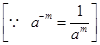= 1/9

(ii) (-4)-2 = (1/-4)2= 1/16

(iii) (1/2)-5 = (2/1)5= 25

= 32

2.  Simplify and express the result in power notation with positive exponent:

(i) (-4)÷(-4)8

(ii) (1/23)2

(iii) -(3)4×(5/3)4

(iv) (3-7÷3-10)×3-5

(v) 2-3×(-7)-3

Solution:

(i)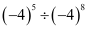= (-4)5/(-4)8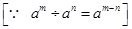= (-4)5-8

= 1/(-4)3

(ii) (1/23)2

= 12/(23)2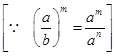= 1/23×2 = 1/26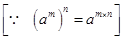(iii) -(3)4×(5/3)4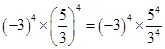= (-1)4×34×(54/34 )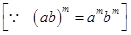= 3(4-4)×54= 30×54 = 54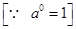(iv)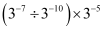=   (3-7/3-10)× 3-5

= 3-7 – (-10) × 3-5= 3(-7+10)×3-5

= 33×3-5

= 3(3+-5)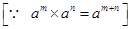= 3-2

=1/32(v) 2-3×(-7) – 3

= (2×-7)-3

(Because am×bm = (ab)m)

= 1/(2×-7)3= 1/(-14)3

3. Find the value of :

(i) (30+4-1)×22

(ii) (2-1×4-1)÷2 – 2

(iii) (1/2)-2+(1/3)-2+(1/4)-2

(iv) (3-1+4-1+5-1)0

(v) {(-2/3)-2}2

Solution:

(i)(30+4– 1)×22 = (1+(1/4))×22= ((4+1)/4 )×22

= (5/4)×22

= (5/22)×22

= 5×2(2-2)= 5×20

= 5×1 = 5(ii)(2-1×4-1)÷2-2

= [(1/2)×(1/4)] ÷(1/4)= (1/2×1/22 )÷ 1/4

= 1/23÷1/4

= (1/8)×(4)

= 1/2

(iii) (1/2)-2+(1/3)-2+(1/4)-2

= (2-1)-2+(3-1)-2+(4-1)-2= 2(-1×-2)+3(-1×-2)+4(-1×-2)= 22+32+42

= 4+9+16

=29

(iv) (3-1+4-1+5-1)0

= 1(v) {(-2/3)-2}2 = (-2/3)-2×2= (-2/3)-4

= (-3/2)4= 81/16

4. Evaluate

(i) (8-1×53)/2-4

(ii) (5-1×2-2)×6-1

Solution:

(i) (8-1×53)/2-4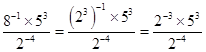=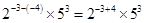= 2×125 = 250

(ii) (5-1×2-2)×6-1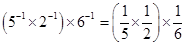= (1/10)×1/6

= 1/60

5. Find the value of m for which 5÷ 5-3 = 55

Solution:

5m ÷ 5-3 = 55

5(m-(-3) ) = 555m+3 =55

Comparing exponents both sides, we get

m+3 = 5

m = 5-3

m = 2

6. Evaluate

(i)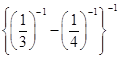(ii)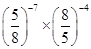Solution:

(i)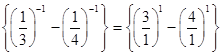= 3-4

= -1

(ii)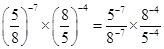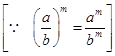=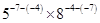=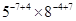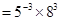=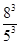=  512/125

7. Simplify.

(i)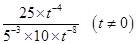(ii)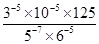Solution:

(i)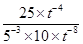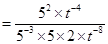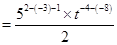=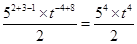=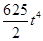(ii)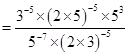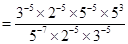=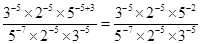=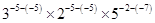=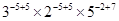=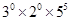= 1×1×3125= 3125

## Exercise 12.2 Page No: 200

1. Express the following numbers in standard form.

(i) 0.0000000000085

(ii) 0.00000000000942

(iii) 6020000000000000

(iv) 0.00000000837

(v) 31860000000

Solution:

(i) 0.0000000000085 = 0.0000000000085×(1012/1012) = 8.5 ×10-12

(ii) 0.00000000000942 = 0.00000000000942×(1012/1012) = 9.42×10-12

(iii) 6020000000000000 = 6020000000000000×(1015/1015) = 6.02×1015

(iv) 0.00000000837 = 0.00000000837×(109/109) = 8.37×10-9

(v) 31860000000 = 31860000000×(1010/1010) = 3.186×1010

2.Express the following numbers in usual form.

(i) 3.02×10-6

(ii) 4.5×104

(iii)3×10-8

(iv)1.0001×109

(v) 5.8×1012

(vi)3.61492×106

Solution:

(i) 3.02×10-6 = 3.02/106 = 0 .00000302

(ii) 4.5×104 = 4.5×10000 = 45000

(iii) 3×10-8 = 3/108 = 0.00000003

(iv) 1.0001×109 = 1000100000

(v) 5.8×1012 = 5.8×1000000000000 = 5800000000000

(vi) 3.61492×10= 3.61492×1000000 = 3614920

3. Express the number appearing in the following statements in standard form.

(i) 1 micron is equal to 1/1000000 m.
(ii) Charge of an electron is 0.000, 000, 000, 000, 000, 000, 16 coulomb.
(iii) Size of bacteria is 0.0000005 m
(iv)  Size of a plant cell is 0.00001275 m
(v) Thickness of a thick paper is 0.07 mm

Solution :

(i) 1 micron = 1/1000000

= 1/106

= 1×10-6

(ii) Charge of an electron is 0.00000000000000000016 coulombs.

= 0.00000000000000000016×1019/1019

= 1.6×10-19 coulomb

(iii) Size of bacteria = 0.0000005

=  5/10000000 = 5/107 = 5×10-7 m

(iv) Size of a plant cell is 0.00001275 m

= 0.00001275×105/105

= 1.275×10-5m

(v) Thickness of a thick paper = 0.07 mm

0.07 mm = 7/100 mm = 7/102 = 7×10-2 mm

4. In a stack there are 5 books each of thickness 20 mm and 5 paper sheets each of thickness 0.016 mm. What is the total thickness of the stack?

Solution:

Thickness of one book = 20 mm

Thickness of 5 books = 20×5 = 100 mm

Thickness of one paper = 0.016 mm

Thickness of 5 papers = 0.016×5 = 0.08 mm

Total thickness of a stack = 100+0.08 = 100.08 mm

= 100.08×102/102 mm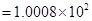mm

Access Class-8 Chapter-wise Solutions: